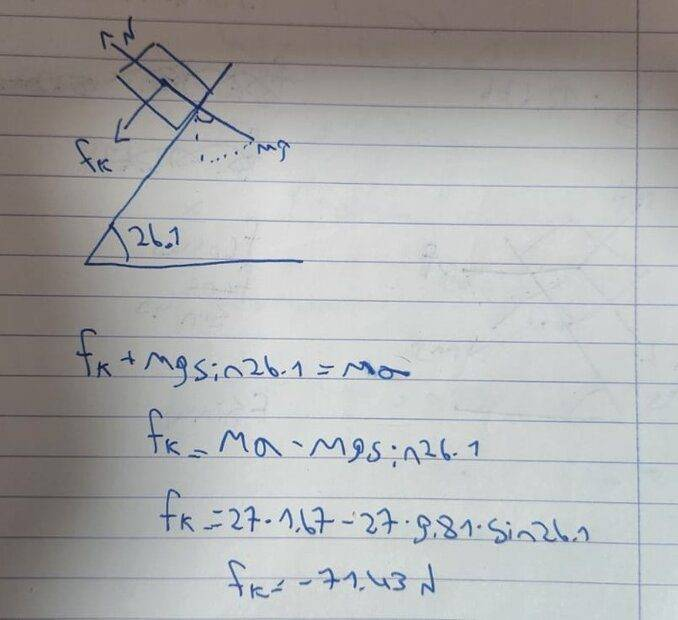# Friction on a box in the bed of a pickup truck accelerating up an incline

• yesmale4
You need to figure out which force is accelerating the block upward and use that to determine the direction of the friction.

#### yesmale4

Homework Statement
A box of mass 27 kg is in the bed of a truck on a hill that is inclined at an angle of 26.1° above the horizontal. The tailgate of the truck is open. The truck is currently accelerating up the hill with a magnitude of 1.67 m/s2. What is the magnitude of the friction force from the truck on the box in this situation?
Relevant Equations
F=ma
hey i would like to get some help with this question i try to answer it but it was not correct i like to know what i did wrong and how i can fix it:Hi,
Which way is the friction force working ?

BvU said:
Hi,
Which way is the friction force working ?
i assume that because of the truck is accelerating up the hill the friction is In the opposite direction like i drew it

yesmale4 said:
i assume that because of the truck is accelerating up the hill the friction is In the opposite direction like i drew it
So which force is accelerating the box upwards so that it does not fall off the truck?

Orodruin said:
So which force is accelerating the box upwards so that it does not fall off the truck?
i see what you mean but i don't know what the answer is i want to say the friction but its pointed down but on the other hand i have to put the friction down becuase its need to be In the opposite direction of truck acceleration so I really don't know

yesmale4 said:
i have to put the friction down becuase its need to be In the opposite direction of truck acceleration
Why?

yesmale4 said:
i see what you mean but i don't know what the answer is i want to say the friction but its pointed down but on the other hand i have to put the friction down becuase its need to be In the opposite direction of truck acceleration so I really don't know
Check this: if the slope is zero, which way does the friction work ?

Orodruin said:
Why?
I remember we were told that the direction of friction was opposite to the direction of motion

I mean, the real problem here though: You have assumed that the block accelerates in the same direction as you have drawn the frictional force, ie, downwards. In order to not fall off, the block needs to accelerate upwards.

BvU said:
Check this: if the slope is zero, which way does the friction work ?
if the truck moving forward then the friction work backwards

yesmale4 said:
I remember we were told that the direction of friction was opposite to the direction of motion
No. That is a misinterpretation. The friction acts opposite to the direction of relative motion between the object itself and the surface it is sliding on or, in the case of zero relative motion as in this case, opposite to the direction the object would otherwise be accelerated in.

Orodruin said:
I mean, the real problem here though: You have assumed that the block accelerates in the same direction as you have drawn the frictional force, ie, downwards. In order to not fall off, the block needs to accelerate upwards.
i understand maybe you can show me how i should draw it?

yesmale4 said:
i understand maybe you can show me how i should draw it?
You can draw the friction any direction you want. If you happen to draw it in the wrong direction it will just come out negative and correct you automatically. Your major issue, as I said, is assuming the block accelerates downward.